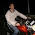### Drunk Guests

Source: Problem 1.27 from the book "Heard from the Street"

Problem:

A very large number, N, of people arrive at a convention. There are exactly N single rooms in the hotel where the convention takes place. Each guest is given a numbered key for a specific room. Before they even go upstairs, they are all invited to a large party in the banquet hall. To gain admittance to the hall, they have to give up their keys to a doorman. At the end of the evening, the guests are not sober enough to recall their room numbers, so the doorman simply hands out the keys randomly. Each guest ends up spending the night in a random room.

What is the probability that at least one guest ends up in a room he or she was originally assigned?
What is the expected number of guests who end up in a room in which they were originally assigned?

Update(18/02/10):
Solution:
Posted by Vivek Chaurasiya (Software Eng Symantec & CSE, IITR Alumnus) in comments!! Explanation and a different solution by me in comments!!

1.This problem is a simple extension of concept known as "Principle of De-rangement' which states that [none of the elements of the set appears in its original position]:

p(A u B u C) = p(A)+p(B)+p(C) - p(AB) - p(AC) - p(BC) = p(ABC)

Once can foresee (or by induction) that if we write such formula for 4 events or N events, then the minus sign will vary alternately.

In our problem, if we find out p(123....N) (say Q) then the required probability =
(1 - Q)

For e.g. if N=5;

Total No. of permutations = 120
No. of permutations in which no person goes to his assigned room = 44 (deduce this from above stated principle )

So, required prob = 1 - (44/120)

Hint:

44 = 5! ( 1 - 1/1! + 1/2! - 1/3! + 1/4! - 1/5!)

2.Correction above:
~~~~~~~~~~~~~~~~~~~~~~~
p(A u B u C) = p(A)+p(B)+p(C) - p(AB) - p(AC) - p(BC) + p(ABC)
~~~~~~~~~~~~~~~~~~~~~~~

About Q2: What is the expected number of guests who end up in a room in which they were originally assigned?

say D(n): no. of derangements of n objects

So, E( guests who end up in a room in which they were originally assigned ) = U (say)
U = E(1) + E(2) + E(3) ....... ....... . . E(n)

Now, E(j) = j . p(j)

p(j) = probability that j persons goto their respective rooms while others dont.
= nCj * D(j)/ n!

U = Summation (j=1 to n) [ j * p(j) ]

Concept part done till here. Rest all simplification steps will be guided by mathematics and series manipulations.

Finally, U comes out to be = 1 (so, one can verify their solving)

3.@VKC (Vivek Kumar Chaurasiya)
Thanx for the solutions.

Regarding the first part:
Correct solution. Thanx a lot. I had not been able to solve it.
Just of clarify things, reposting:

Considering 3 guests
p(A u B u C) = p(A)+p(B)+p(C) - p(AB) - p(AC) - p(BC) + p(ABC)

p(A) means A spends the night in his room.

p(A) = p(B) = p(C) = 1/3 = 1/n

P(AB) = P(BC) = P(CA) = 1/n.1/(n-1)

and so on

So, p(A u B u C) = n*1/n - nC2 * 1/n*1/n-1 + + nCn 1/n*1/(n-1)*1/(n-2)

So,
p(A1 u A2 u .. .. An) = 1 - 1/2! + 1/3! -1/4! and so on

So, for n=5,
p(A1 u A2 u .. .. A5) = 1-1/2! + 1/3! -1/4! + 1/5!

Required probability = (120-60+20-5+1)/120 = 76/120

Also, as n approaches infinity, Required probability = 1-(1/e) :)

Regarding the second part: Answer 1 is correct and there is a simpler method. U = P1 + P2 + .. Pn

where Pi is one if guest i goes to the correct room and zero otherwise

E(U) = E(P1 + P2 +.. Pn)
By linearity of expectation,
E(U) = E(P1) + .. E(Pn) = 1/n*n = 1
:)

Thanx